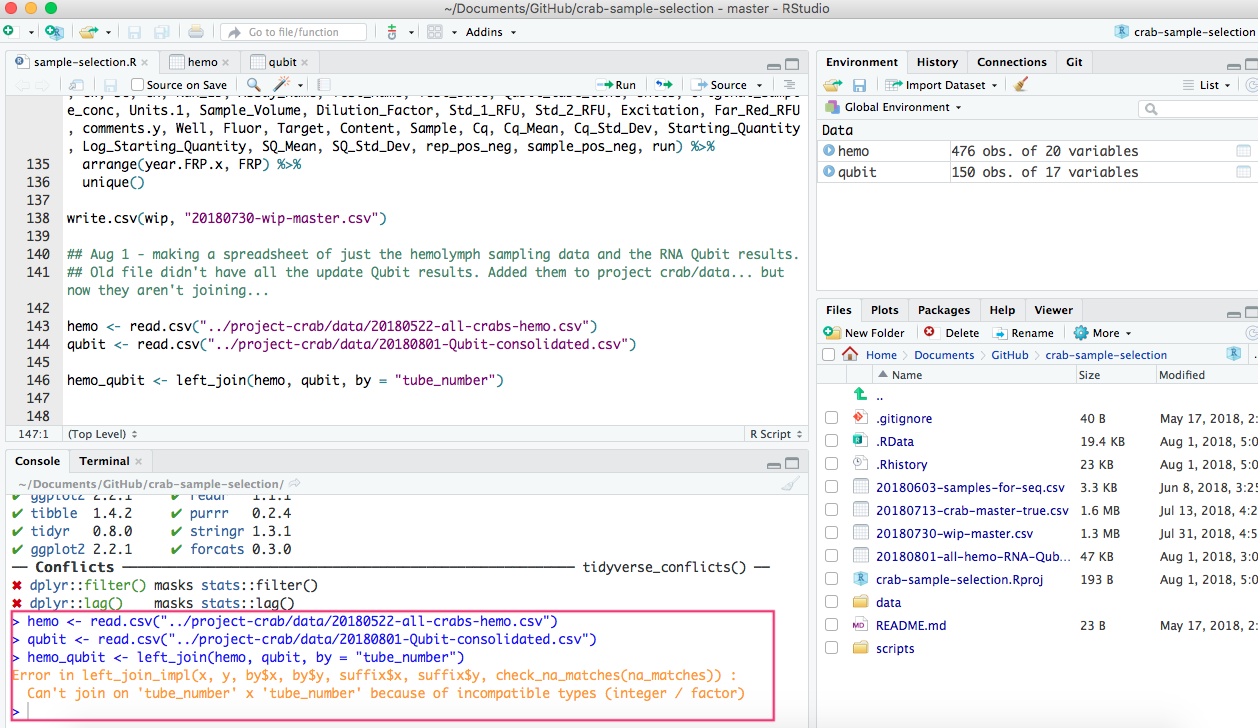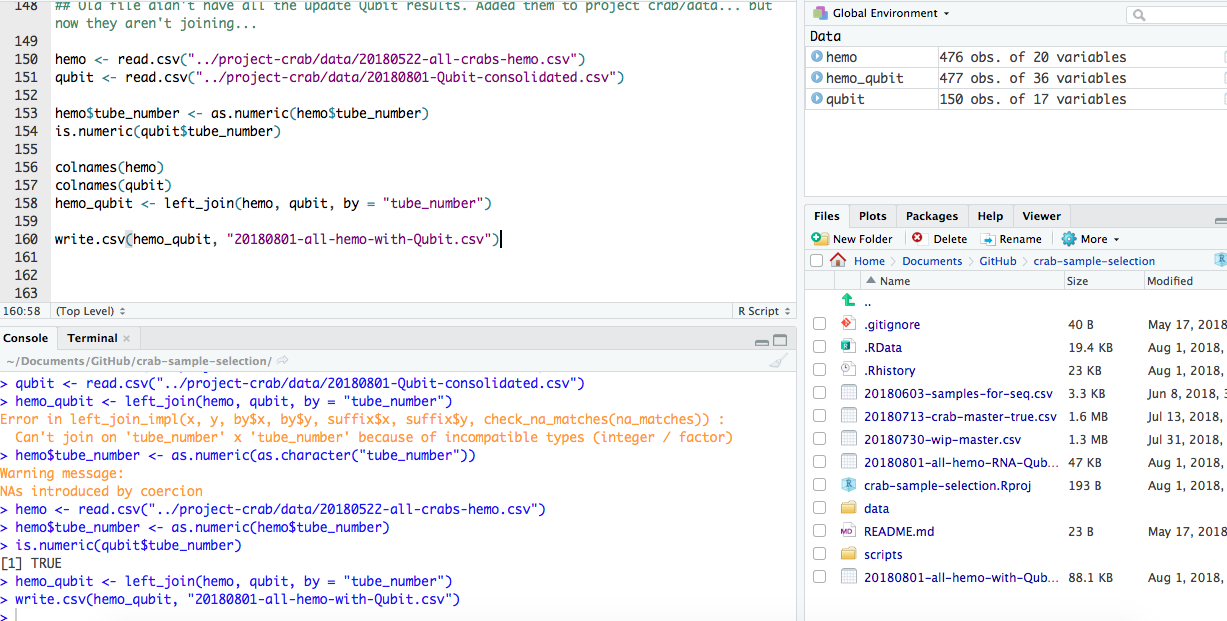Today I noticed that the code Yaamini showed me yesterday to change the “tube_number” column values in the 20180522-all-crabs-hemo.csv from Factor to numeric ended up changing the tube numbers, which meant that the spreadsheet I made yesterday was wrong. So, Sam taught me how to make the “tube_number” column from Factor to numerics without changing the value, as described below.

## What I did yesterday with Yaamini:

(GitHub Issue #334)
The files:

The problem:
`left_join` based on the column “tube_number” wasn’t working due to the fact that 20180522-all-crabs-hemo.csv had the values as Factors, while 20180801-Qubit-consolidated.csv had them as integers.What we thought was the solution:It was not.

After looking at joined the file more closely today, I realized that the tube numbers in the column “tube_numbers” were not the same as those in the “Uniq_ID” column. The unique ID was created by concatenating the FRP number, tube number, and sample day for each individual sample.
Example:
6125_503_26
Where:
6125 = FRP (unique to each crab)
503 = sample tube number (unique to each sample)
26 = sample day (of which there are 3: 9, 12, and 26)

GitHub Issue #337

After looking more closely at the code and going through it very slowly, I realized the issue happened when I did the code for changing the “tube_number” column in 20180522-all-crabs-hemo.csv from Factors to numbers.

Sam responded with:
“Oh, I see what’s happening! This is an issue with converting a factor to numeric. As it turns out, factors are actually number representations. So, when you convert a factor to numeric, you’re seeing the numeric representation of the value that was in a given field. It sounds convoluted (and, sorta is), but makes some sense if you read up on it.”

And after some trial and error and some SERIOUS help from Sam (he told me what to type), this ended up being the solution: